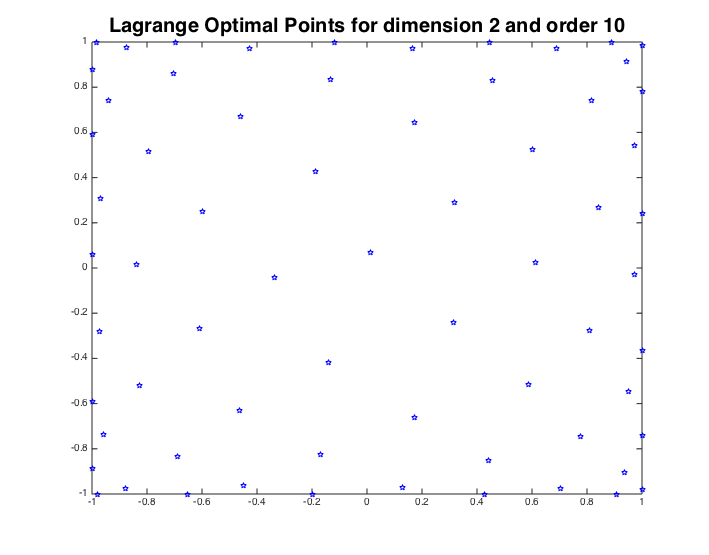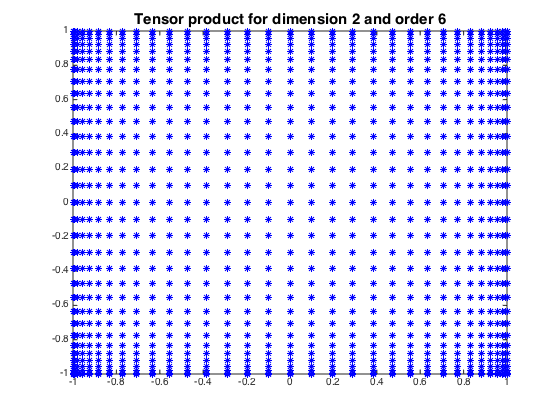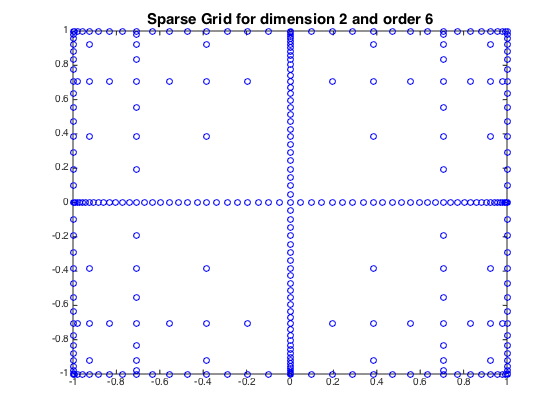Training Sets

Lagrange Optimal points

Our first candidate for a training set are the Lagrange optimal points which are based on the Lagrange characteristic polynomials.

Let$\Pi_\beta^K=\{\chi:\mathbb{R}^K\to \mathbb{R}:\chi \text{ polynomial with } \text{deg}(\chi)\leq \beta\}$ be the set of polynomials on$\mathbb{R}^K$ with total degree at most$\beta$ and let$q=\dim(\Pi_\beta^K)=\binom{\beta+K}{K}$. For$X_{\beta,K}=\{\xi_j\}_{j=1}^q\subset\Gamma$ consider$\{l_n\}_{n=1}^q$ , the Lagrange characteristic polynomials, defined so that$l^n(\xi_j)=\delta_{nj}, n,j\in\{1,\ldots,q\}$ which form a basis of$\Pi_\beta^K$. They can be written in terms of Vandermonde determinants,$l^n(x)=\frac{\det[V(\xi_1,\ldots,\xi_{n-1},x,\xi_{n+1},\ldots, \xi_q)]}{\det[V(\xi_1,\ldots,\xi_q)]}, \quad n=1,\ldots,q,$

where$V\in\mathbb{R}^{q\times q}$ is such that$(V(\lambda_1,\lambda_2, \ldots,\lambda_q))_{jn}=l^n(\lambda_j)$. Recall that the Lebesgue constant$\lambda_{q,K}$ of$X_{\beta,K}$ is defined by,$\lambda_{q,K}:= \max_{x\in\Gamma} \sum_{n=1}^q|l^n(x)|.$

We are interested in the solutions of the following optimization problem,$X^*_{\beta,K}=\underset{\{\xi_j\}_{j=1}^q}{\arg\min}\max_{x\in\Gamma} \sum_{n=1}^{q}|l^{n}(x)|.\quad (3)$

These solutions constitute the definition of the Lagrange optimal points.Clenshaw-Curtis points

Consider the$N$-th degree Chebyshev polynomial$T_N(x)$ defined by$T_N(cos(\theta))=cos(N\theta)$. The Gauss-Chebyshev-Lobatto points (of degree$N$) are the extrema in$[-1,1]$ of$T_N(x)$. They are given by the explicit formula:$x_n=cos\bigg(\frac{n\pi}{N}\bigg) \smallskip n=0,\ldots,N.$

The Clenshaw-Curtis points are the nested subsequence of such points with$N(i)=2^{i-1}+1$$(i.e. \{x_n\}_{1 \le n \le N(i)} \subset \{x_n\}_{1 \le n \le N(i+1)})$

A property of the Clenshaw-Curtis points that can be easily deduced from the explicit formula above is the asymptotic (for$N$ large) clustering of points near the boundary (i.e. near -1 and 1).Sparse Grids

Previously we discussed the one dimensional Clenshaw-Curtis points and now we would like to go to an arbitrary dimension$K >$ 1. As soon as we try that we run into the Curse of Dimensionality: If we took the full tensor product of$N$ one dimensional points we end up with$N^K$ points in$K$ dimensions which is far too big a number for numerical schemes. The technique of Sparse Grids allows us to overcome this problem.

We start off with$N$ one dimensional points$\{x_1,\dots x_N\}$. We would like to build a collection$H^{s}_{K,q}$ of$K$-dimensional points, our sparse grid. Let$i=(i_1,\ldots,i_K)$ be a multi-index and consider the norm$|i|_{l_1}= \Sigma_{k=1}^{K}i_k$ and let$q \geq K.$

Take a nested family of collections of points$\{\Theta^{j}\}$ so that$\Theta^{j} \subset \{x_1,\dots x_N\}$ for all$j$,$\Theta^{j} \subset \Theta^{l}$ if$l>j$ and$|\Theta^{j}|=2^{j-1}+1.$

Now define the sparse grid in$K$ dimensions as follows,$H_{K,q}^s= \underset{q-K+1 \leq |i|_{{l}_{1}} \leq q}{\bigcup} \big( \Theta^{i_1} \times \ldots \times \Theta^{i_K} \big).$

One should note that even though the actual points in the sparse grid depend on how we chose the nested family$\{\Theta^j\}$, the cardinality of the sparse grid is independent of this choice.Other points that we have used as training sets are :

• Uniform Grid points.
• Random points.